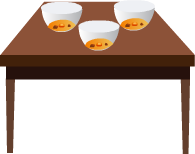# Multiplying Fractions with Whole Numbers

Rate Lesson 0 stars
Common Core
• Lesson size:
Message preview:
Someone you know has shared lesson with you:

To play this lesson, click on the link below:

https://www.turtlediary.com/lesson/multiplying-fractions-with-whole-numbers.html

Hope you have a good experience with this site and recommend to your friends too.

Login to rate activities and track progress.
Login to rate activities and track progress.
CCSS.Math.Content.4.NF.B.4CCSS.Math.Content.5.NF.B.4

Multiplying fractions with whole numbers is much easier than adding fractions.

Let us learn this by following examples :

## Example 1

If we have four children and each has two cookies, how many cookies are there in all?If we multiply 4 x 2, we find that there are 8 cookies total.

But what if we have four children and each has
2
3

Just like with whole numbers, we can use multiplication to solve a problem like this.

Multiply the numerator by the whole number, and leave the denominator the same.

We need to multiply 4 x
2
3
First, multiply the numerator by the whole number.

4 x 2 = 8

Remember, the denominator stays the same, so our answer is:

8
3

Since the numerator is larger than the denominator, you can tell that this fraction is bigger than one.

You can restate it as 2
2
3
.

Here is how we did it. To change an improper fraction to a mixed number, you must divide the numerator by the denominator. Then, the mixed number takes the following form :

• The answer becomes the whole number
• The remainder becomes the numerator
• The denominator stays the same## Example 2

There are three bowls of soup on a table. Each bowl is
1
5
full. How much soup is there in total?We need to multiply 3 x
1
5
.

3 x 1
5
=
3
5

There is
3
5
of a bowl soup in total.
Summary
• Fractions represent parts of whole numbers
• Numerator (number on top) represents how many parts of the whole you have
• Denominator (number on the bottom) represents how many equal parts the whole is divided into.
• Multiplying Fractions by a Whole Number: Multiply the numerator by the whole number, and leave the denominator the same.

## More Fractions Lessons

Become premium member to get unlimited access.